# 1st PUC Computer Science Model Question Paper 2 with Answers

Students can Download 1st PUC Computer Science Model Question Paper 2 with Answers, Karnataka 1st PUC Computer Science Model Question Papers with Answers helps you to revise the complete Karnataka State Board Syllabus and score more marks in your examinations.

## Karnataka 1st PUC Computer Science Model Question Paper 2 with Answers

Time: 3 Hours 15 Minutes
Max. Marks: 70

PART – A

I. Answer the following questions. (10 × 1 = 10)

Question 1
What is the function of ALU?
ALU unit performs all the arithmetic calculations and logical operations involved in a program.

Question 2.
What are ergonomic keyboards?
It is a special type of keyboard that allows typing without much strain and bending at the wrists. It reduces muscle and finger pain while typing.

Question 3.
What is the important aspect of the design of a solution?
The most important aspect of developing a solution is, developing the logic to solve the specific problem.

Question 4.
Give the syntax of cin statement.
Syntax:
cin >> variable.

Question 5.
What is meant by stream extraction?
cin is used in conjuction with >> operator, known as extraction or get from operator.

Question 6.
What is meant by a compound statement?
A compound statement is a grouping of statements enclosed between the pair of braces ({}) in which, each individual statement ends with a semi-colon.

Question 7.
What is zero based indexing?
If ‘i’ is the element, given its index number would be i-1 and it is called zero based indexing.

Question 8.
What is meant by calling a function?
To use a function, user will have to call or invoke that function. It helps to execute the function and perform the process of a function.Question 9.
Define formatting in wordprocessor.
Formatting is the process of changing the attribute of a text document.

Question 10.
What is a value c Ml in spreadsheet?
A cell that contains numbers with or without a + or – symbol is called a value cell.

PART – B

II. Answer any Five of the following questions. (5 × 2 = 10)

Question 11.
Expand the term ENIAC and EDVAC.

• Electronic Numerical Integrator and Calculator.
• Electronic Discrete Variab’e Automatic Computer.

Question 12.
Give one example each for hard copy and soft copy devices.
Printer is a hardcopy device and Visual Display Unit is a soft copy device.

Question 13.
What is meant by machine dependent language and machine independent language?

• Set of programs that run only on specific types of CPU are called machine dependent.
• Programs that can be run on a variety of different types of computers are called machine independent.

Question 14.
What is testing and debugging?

1. Testing is the process of checking the program logic, by providing selected sample data and observing the output for correctness.
2. The process of detecting the errors and correcting them in a program is called debugging.

Question 15.
The object-oriented programming provides improved software development productivity over traditional procedure based programming techniques, because of the factors like modularity, extensibility and reusability.

Larger program size:
Object-oriented programs typically involve more lines of code than procedural programs.

Question 16.
What are linear and non-linear data structures?

• When data elements are arranged in a sequential manner, it is called as linear data structure.
• When the data elements are arranged non-sequentially, it is called as non linear data structure.Question 17.
Write the names of any two C++ header files.

• iostream.h
• iomanip.h

Question 18.
Write the structure definition to store name of the student, register number, class and Show how is the structure initialized? Give an example.PART – C

III. Answer any Five of the following questions. (5 × 3 = 15)

Question 19.
What is OMR and where is it used?
Optical Mark Reader: It is an input device that can detect marks made in predetermined positions on a pre-printed paper called OMR sheet. An optical mark reader shines a light beam onto the input document and is able to detect the marks because less light is reflected back from them, than from the unmarked area. It is used in grading test papers.

Question 20.
What is radix or base of a number system? Give the radix of different number systems.
The radix or base is the number of unique digits, including zero that a positional numeral system uses to represent numbers.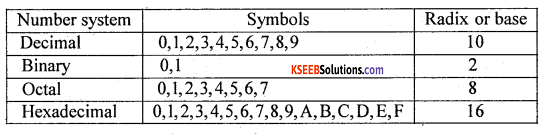Question 21.
What is a multitasking operating system? Give an example.
In a multitasking operating system several applications may be simultaneously loaded and used in the memory. While the processor handles only one application at a particular time, it switches between the applications and simultaneously executes each application. For example, Windows operating system.

Question 22.
What are sequential constructs, selective construct an iterative construct?
1. Sequential construct:
The ability to execute the program statements one after another in sequence is called sequential construct.

2. Selective construct:
It is the process of selecting certain set of statements based on a requirement for execution.

3. Iterative construct:
It is the process of the execution of certain set of statements repeatedly until a requirement is satisfied.

Question 23.
Define character set, integer constant, and floating-point constants.
Character set is a set of symbols that a programming language identifies and uses in writing data and instructions in a programming language. Integer constants are whole numbers without any fractional part. Floating-point constants are numeric values that contain a decimal point and can contain exponents also.

Question 24.
Explain manipulators.
1. A manipulator in C++ is used to control the formatting of output and/or input values.

2. Manipulators can only be present in Input/Output statements. The ‘end!’ manipulator causes a newline character to be output.

3. ‘end1’ is defined in the header file and can be used as long as the header file has been included.Question 25.
How can we input the values into a one-dimensional array?Question 26.
What is array of structures? Give an example.
The array of structures is a collection of array elements, in which each element is a structure ‘ in an array.
Example:
struct student st[ 100];

PART-D

IV. Answer any Seven of the following questions. (7 × 5 = 35)

Question 27.
Briefly explain the characteristics of a computer.
1. Speed:
Computers are fast in doing calculations. The speed of the computer is measured in terms of millions of instructions per second (MIPS).

2. Storage Capacity:
Computers come with a large amount of memory. They can hold lot an of data. Computers can select and display a particular piece of information from a very large amount of data within a short time.

3. Diligence:
After doing work for some time, humans become tired but computers do not become tired. They work continuously. In fact, some computers which control telephone exchanges are never shut down. This is called diligence.

4. Accuracy:
The results that the computers produce are accurate provided data and programs are reliable.

Question 28.
Explain binary subtraction using 1’s complement in brief.
Subtraction with one’s complement:
A 5-bit binary number 011012 (910) is subtracted from another 5-bit binary number 010012 (1310) by converting 010012 to its negative equivalent in 1 ’s complement and adding this value to 011012.

1. First, 010012 is converted to its negative equivalent in 1’s complement.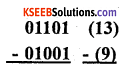2. To do this all the 1’s to 0’s are changed and 0’s to 1’s. The most-significant digit is now 1 since the number is negative.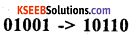3. Next, the negative value computed is added to 0110.12. This gives a result of 1000112.4. This addition has caused an overflow bit. Whenever there is an overflow bit in 1 ’s complement, this bit is added to the sum to get the correct answer. This gives the final answer as 001002 (or 410).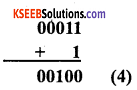Question 29.
Give the list of statements which can be used and which cannot be used in structured programming.
List of statements that can be used:

• sequence of sequentially executed statements.
• conditional execution of statements
• iteration execution statements.

List of statements that cannot be used:

• goto statement
• break or continue statement
• multiple exit points.Question 30.
What are unary operators, binary operators, relational operators, logical operators, and bitwise operators?
1. An operator that takes only one operand to perform some operation is called a unary operator.

2. The operator which takes two operands to perform some operation is called a binary operator.

3. The operators which perform operation of relation between two operands are called relational operators.

4. The operators which perform combine or negate the expressions that contain relational operators are called logical operators.

5. Bit manipulation operators manipulate individual bits within a variable. Bitwise operators modify variables considering the bit patterns that represent the values they store.

Question 31.
What are selection statements? Write the syntax for different types of selection statements.
Selection statements are used to execute certain blocks of statements by evaluating the condition.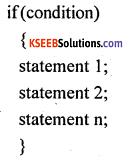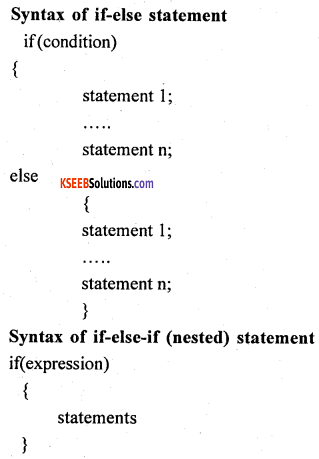Question 32.
Explain while loop structure with an example.
A while loop statement repeatedly executes a statement or sequence of statements written within the flower brackets as long as a given condition returns the value ‘true’.
Syntax:
The syntax of a while loop in C++ is: while(condition)
{
statement(s);
}
Here the condition may be any expression, and true for any non zero value. The loop iterates while the condition is true. When the condition becomes false, program control passes to the line immediately following the loop. During the first attempt, when the condition is tested and the result is false, the loop body will be skipped and the first statement after the while loop will be executed.
Example: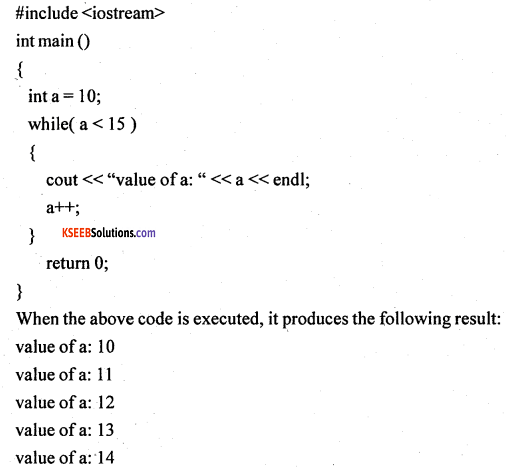Question 33.
Write a program to count the number of vowels and consonants in a string.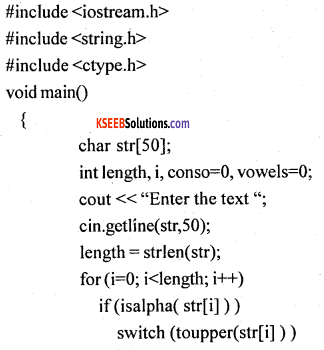Question 34.
Explain function with argument and no return value with an example.
The calling function main() gives the function call to the called function by passing arguments or values. Then the called function takes the values and performs the calculations and itself gives the output but no value is sent back to the calling function.
For example:In the above example, sum() is a user defined function. main() is a calling function which gives a function call sum (a, b) with actual arguments a, b. The copy of values of a and b are sent to formal arguments x and y in the called function sum(). The function sum() performs addition and gives the result on the screen. Here it can be observed that no value is sent back to the calling function main(). These kinds of functions are called “Function with argument but no return values”.

Question 35.
The different uses of spreadsheet are as follows:

• Performing basic mathematical operations such as adding columns and rows of figures.
• Finding values such as profit or loss.
• Calculating repayment plans for loans or mortgages.
• Finding the average, maximum or minimum values in a specified range of data.
• Graphing or charting data to assist users in identifying data trends.
• Sorting and filtering lata to find specific information.
• Creation of simple lists and tables of alphabetic or numerical data
• Creation and manipulation of simple (flat-file) databases

Question 36.
Define the following

1. workbook
2. cell
4. active cell
5. cell pointer

1. workbook:
A workbook is a set of electronic spreadsheets.

2. cell:
The intersection of a row and a column in a spreadsheet is called a cell.

A cell address is a unique ID given to a cell, represented by column name and row name.

4. active cell:
The cell that is currently open and ready for editing is called the active cell.

5. cell pointer:
Cell pointer is a rectangular box that appears in the worksheet and is used to make an entry in a cell.Question 37.
Explain different services of internet.
The different services of internet are email, chat, newsgroup, FTP, WWW, etc.,
Email:
It is a system for exchanging written, voice and video messages through a computer network. Some users of email are as follows:

• Messages can be sent 24×7 at any convenient time that suits the user.
• Messages reach recipient’s mailbox within minutes.
• Delivery of messages can be confirmed.

1. Chat:
It is an online communication between two users through computer on internet. Once a chat has been initiated, either user can enter text by typing on the keyboard and the entered text will appear on the other user’s monitor. Most networks and online services offer a chat facility.

2. Bulletin Board:
It is an electronic message center where people can post public messages. Messages can be placed on the computer so people can leave them for other people to read and see and later erase them when not required. For example, it is used to advertise things to buy or sell, announce events, or provide information.

3. FTP:
It is a standard network protocol used to exchange and manipulate files over a TCP/IP based network, such as the Internet. FTP is most commonly used to download a file from a server using the Internet or to upload a file to a server.

4. Telnet:
Telnet stands for ‘telecommunications network’, and was built to be a form of remote control to manage mainframe computers from distant terminals. Telnet is a text-only protocol and the user cannot see graphics or fancy images while working with Telnet, nor will they be able to transfer files with Telnet. Telnet is used to communicate with other computers and machines in a text-based manner.

error: Content is protected !!